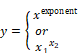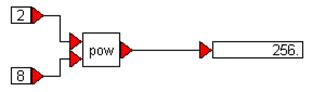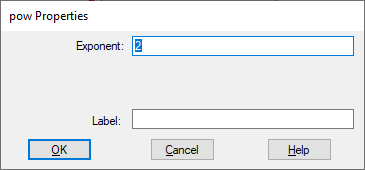## powBlock Category: Arithmetic

Description: The pow block creates an output signal based on the value of the input signal raised to the power of a specified exponent. The inputs can be real or complex scalars, vectors, or matrices. When the input is a vector or matrix, the pow block computes the output on an element-by-element basis. If the input signal is negative, the exponent must be an

The pow block is useful for solving equations of the type y = xz. Do not use the pow block to compute matrix dot products, such as Y = A2, where the dot product is implied. Instead use the dotProduct block.

By adding an input connector tab to the pow block, you can specify an external exponent parameter to override the block’s exponent parameter. For example:This diagram raises two to the eighth power. The display block verifies the results. The main advantage of setting the exponent externally is that the value of the exponent can be varied dynamically as the simulation progresses.Exponent: Specifies the power to which the input signal is raised. If the input signal is negative, the exponent must be an integer; otherwise, a domain error occurs. The default is 2. You can also enter a value as a C expression.

Label: Indicates a user-defined block label that appears when View > Block Labels is activated.